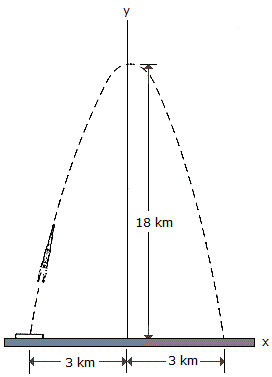# Engineering Mechanics - Kinematics of Particle (KOP) - Discussion

### Discussion :: Kinematics of Particle (KOP) - General Questions (Q.No.32)

32.For a short time the missile moves along the parabolic path y = (18 - 2x2) km. If motion along the ground is measured as x = (4t - 3) km, where t is in seconds, determine the magnitudes of the missile's velocity and acceleration when t = 1 s.

 [A]. v = 5.66 km/s, a = 4.0 km/s2 [B]. v = 16.49 km/s, a = 64.0 km/s2 [C]. v = 16.00 km/s, a = 22.6 km/s2 [D]. v = 4.00 km/s, a = 16.03 km/s2

Explanation:

No answer description available for this question.

 Hermes said: (Aug 16, 2021) y = 18 - 2x^2, = 18 -2(4t-3)^2, = -32t^2 + 48t. Vy = dy/dt, = -64t + 48. ay = dv/dt, = -64. x = 4t -3. Vx = dx/dt. = 4, ax = dx/dt. = 0. when t = 1s. Vy = -16 km/s^2, Vx = 4 km/s^2. v = √(Vy^2 + Vx^2), = 16.49 km/s^2. ay = -64 km/s^2. ax = 0 km/s^2, a = √(ax^2 + ay^2), = 64 km/s^2.